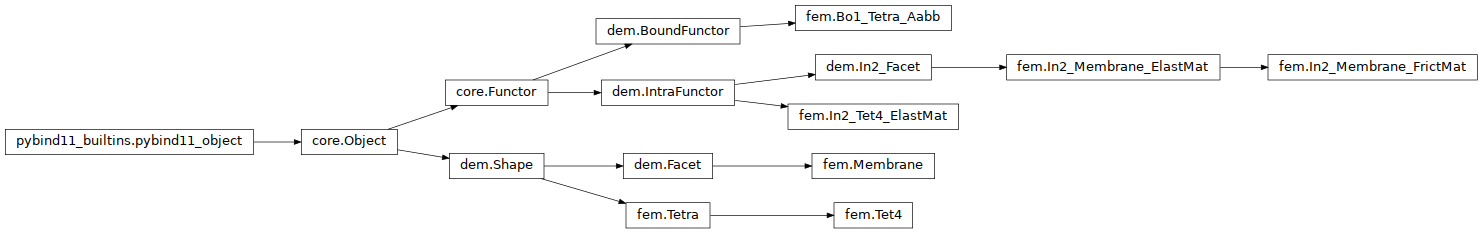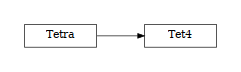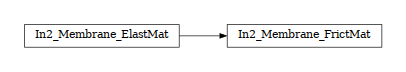# woo.fem¶## Tetra¶class woo.fem.Tetra(*args, **kwargs)

Tetra (triangle in 3d) particle.

1. __init__(self: woo.fem.Tetra) -> None

2. __init__(self: woo.fem.Tetra, *args, **kwargs) -> None

canonicalizeVertexOrder(self: woo.fem.Tetra) None

Order vertices so that signed volume is positive.

getCentroid(self: woo.fem.Tetra) _wooEigen11.Vector3

Return centroid of the tetrahedron

getVolume(self: woo.fem.Tetra)

Return volume of the tetrahedron.

static make(vertices, fixed=True, wire=True, color=None, highlight=False, mat=<function defaultMaterial>, visible=True, mask=3, __class=<class 'woo.fem.Tetra'>)

Create tetrahedral particle

### Tet4¶

class woo.fem.Tet4(*args, **kwargs)

4-node linear interpolation tetrahedron element with best-fit co-rotated coordinates.

1. __init__(self: woo.fem.Tet4) -> None

2. __init__(self: woo.fem.Tet4, *args, **kwargs) -> None

node(= None)

Local coordinate system

[type: shared_ptr<Node>, read-only in python]

refPos(= MatrixX())

Reference nodal positions in local coordinates

uXyz(= VectorX())

Nodal displacements in local coordinates

KK(= MatrixX())

Stiffness matrix

[type: MatrixXr, not shown in the UI, read-only in python]

EB(= MatrixX())

$$E B$$ matrix, used to compute stresses from displacements.

[type: MatrixXr, not shown in the UI, read-only in python]

ensureStiffnessMatrix(self: woo.fem.Tet4, young: float, nu: float) None

Ensure that stiffness matrix is initialized; internally also sets reference configuration. The young parameter should match woo.dem.ElastMat.young attached to the particle.

getStressTensor(self: woo.fem.Tet4) _wooEigen11.Matrix3
static make(*args, **kw)

Create tetrahedral particle

reset(self: woo.fem.Tet4) None
setRefConf(self: woo.fem.Tet4) None

Set the current configuration as the reference one

update(self: woo.fem.Tet4) None

Update current configuration; creates reference configuration if not existing

## In2_Membrane_ElastMat¶class woo.fem.In2_Membrane_ElastMat(*args, **kwargs)

Apply contact forces and compute internal response of a Membrane. Forces are distributed according to barycentric coordinates when bending is enabled; otherwise forces are distributed equally (thirds) to all nodes, to avoid contacts punching through the mesh which has no bending resistance. This can be overridden by setting applyBary, in which case forces will be always applied weighted by barycentric coords.

Note

If your particles are made of ~:obj:woo.dem.FrictMat, use In2_Membrane_FrictMat instead, if you run into ambiguous dipatch errors.

1. __init__(self: woo.fem.In2_Membrane_ElastMat) -> None

2. __init__(self: woo.fem.In2_Membrane_ElastMat, *args, **kwargs) -> None

contacts(= True)

Apply contact forces to facet’s nodes (FIXME: very simply distributed in thirds now)

[type: bool]

nu(= 0.25)

Poisson’s ratio used for assembling the $$E$$ matrix (Young’s modulus is taken from ElastMat). Will be moved to the material class at some point.

[type: Real]

thickness(= nan)

Thickness for CST stiffness computation; if NaN, try to use the double of Facet.halfThick.

[type: Real]

bendThickness(= nan)

Thickness for DKT stiffness computation; if NaN, use thickness.

[type: Real]

bending(= False)

Consider also bending stiffness of elements (DKT)

[type: bool]

applyBary(= False)

Distribute force according to barycentric coordinate of the contact point; this is done normally with bending enabled, this forces the same also for particles without bending.

[type: bool]

rotIncr(= False)

Compute nodal rotation incrementally (by integration of angular velocities) rather than by subtracting from reference rotations (the advantage of incremental is that it is numerically stable even for huge rotations, but perhaps less precise).

[type: bool]

### In2_Membrane_FrictMat¶

class woo.fem.In2_Membrane_FrictMat(*args, **kwargs)

Workaround for current dispatching mechanism limitations so that membrane with woo.dem.FrictMat is not matched by woo.dem.In2_Facet.

1. __init__(self: woo.fem.In2_Membrane_FrictMat) -> None

2. __init__(self: woo.fem.In2_Membrane_FrictMat, *args, **kwargs) -> None

## Bo1_Tetra_Aabb¶

class woo.fem.Bo1_Tetra_Aabb(*args, **kwargs)

Creates/updates an Aabb of a Tetra.

1. __init__(self: woo.fem.Bo1_Tetra_Aabb) -> None

2. __init__(self: woo.fem.Bo1_Tetra_Aabb, *args, **kwargs) -> None

## In2_Tet4_ElastMat¶

class woo.fem.In2_Tet4_ElastMat(*args, **kwargs)

Apply contact forces and compute internal response of a Tet4.

1. __init__(self: woo.fem.In2_Tet4_ElastMat) -> None

2. __init__(self: woo.fem.In2_Tet4_ElastMat, *args, **kwargs) -> None

contacts(= False)

Apply contact forces to Tetra nodes (not yet implemented)

nu(= 0.25)

Poisson’s ratio used for assembling the $$E$$ matrix (Young’s modulus is taken from ElastMat). Will be moved to the material class at some point.

[type: Real]

## Membrane¶

class woo.fem.Membrane(*args, **kwargs)

Facet as triangular element, with 2 translational and 2 rotational degrees of freedom in each node.

Since positions of nodes determine the element’s plane, the $$z$$ degrees of freedom have zero displacements and are condensed away from the KKdkt matrix (force reaction, however, is nonzero in that direction, so the matrix is not square).

Drilling rotations can be computed, but are ignored; this can lead to instability in some cases – wobbly rotation of nodes which does not decrease due to non-viscous damping.

The element is assumed to be under plane-stress conditions.

Mass of the element is lumped in to nodes, but this is not automatized in any way; it is your responsibility to assign proper values of DemData.mass and DemData.inertia.

1. __init__(self: woo.fem.Membrane) -> None

2. __init__(self: woo.fem.Membrane, *args, **kwargs) -> None

node(= <Node @ 0x284c120, at (0, 0, 0)>)

Local coordinate system

[type: shared_ptr<Node>, read-only in python]

refRot(= [])

Rotation applied to nodes to obtain the local coordinate system, computed in the reference configuration. If this array is empty, it means that reference configuration has not yet been evaluated.

refPos(= Vector6(0, 0, 0, 0, 0, 0))

Nodal coordinates in the local coordinate system, in the reference configuration

uXy(= Vector6(0, 0, 0, 0, 0, 0))

Nodal displacements, stored as ux0, uy0, ux1, uy1, ux1, uy2.

Normal load applied to this facet (positive in the direction of the local normal); this value is multiplied by the current facet’s area and equally distributed to nodes.

[type: Real, unit: Pa]

phiXy(= Vector6(0, 0, 0, 0, 0, 0))

Nodal rotations, only including in-plane rotations (drilling DOF not yet implemented)

KKcst(= MatrixX())

Stiffness matrix of the element (assembled from the reference configuration when needed for the first time)

[type: MatrixXr]

KKdkt(= MatrixX())

Bending stiffness matrix of the element (assembled from the reference configuration when needed for the first time).

[type: MatrixXr]

enableStress(= False)

Set to evaluate EBcst and DBdkt when stiffness matricess are being computed. After than, using sigCST and sigDKT will return stresses.

[type: bool]

EBcst(= MatrixX())

CST displacement-stress matrix, for computation of stress tensor (see stressCst).

DBdkt(= MatrixX())

DKT displacement-stress matrix, for computation of stress tensor (see stressDkt.

Warning

This matrix is not computed correctly, therefore also stressDkt returns garbage.

noWarnExcessRot(= False)

Set to disable warning about excessive in-plane rotation. Only do this if you know what you’re doing.

[type: bool]

static make(*args, **kw)

Create facet with given parameters.

Parameters
• vertices ([Vector3,Vector3,Vector3]) – coordinates of vertices in the global coordinate system.

• wire (bool) – if True, facets are shown as skeleton; otherwise facets are filled

See woo.utils.sphere’s documentation for meaning of other parameters.

reset(self: woo.fem.Membrane) None

Reset reference configuration; this forces using the current config as reference when update is called again.

setRefConf(self: woo.fem.Membrane) None

Set the current configuration as the reference one.

stressCst(self: woo.fem.Membrane, glob: bool = False)

Return CST stresses (product of EBcst and uXy), provided that EBcst was computed previously by setting enableStress when building stiffness matrices. The value returned is either $$(\sigma_x,\sigma_y,\sigma_{xy})$$ (local stresses), or Matrix3 representing stress tensor in global coordinates (with glob=True).

stressDkt(self: woo.fem.Membrane) _wooEigen11.Vector6

Return Vector6 of DKT stresses (product of DBdkt and phiXy), see stressCst for conditions; additionaly, bending must have been enabled.

Warning

This function returns nonsense currently and must be fixed!

update(self: woo.fem.Membrane, dt: float, rotIncr: bool = False) None

Update current configuration; create reference configuration if it does not exist.

Tip

Report issues or inclarities to github.